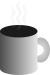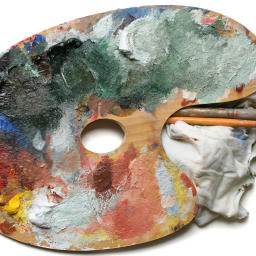#Coffee SpaceListen:

## Colour MixingPreview Image

Sometimes you want to mix two HTML colours together. This Python code was initially written prior to the colour selection algorithm, where colours were manually defined and I needed a way to generate new colours.

Other reasons for wanting such code is to blend HTML colours for a colour picker, or as part of some colour generation tool.

### Code

Here is the code that achieves this (using basic linear mixing):

```0001 # mix_col()
0002 #
0003 # Mix two colours together and return the results hex string.
0004 #
0005 # @param a The first colour.
0006 # @param b The second colour.
0007 # @param ratio A value between 0 and 1 to indicate the mixing ratio.
0008 # @return The resulting colour.
0009 def mix_col(a, b, ratio) :
0010   x = int(a[1:], 16)
0011   x_r = (x & 0xFF0000) >> 16
0012   x_g = (x & 0x00FF00) >>  8
0013   x_b = (x & 0x0000FF)
0014   y = int(b[1:], 16)
0015   y_r = (y & 0xFF0000) >> 16
0016   y_g = (y & 0x00FF00) >>  8
0017   y_b = (y & 0x0000FF)
0018   c_r = int((x_r * ratio) + (y_r * (1 - ratio)))
0019   c_g = int((x_g * ratio) + (y_g * (1 - ratio)))
0020   c_b = int((x_b * ratio) + (y_b * (1 - ratio)))
0021   c = (c_r << 16) | (c_g << 8) | c_b
0022   r = hex(c)[2:]
0023   while len(r) < 6 :
0024     r = "0" + r
0025   return "#" + r```

As you can see, `a` is the first HTML colour, `b` is the second HTML colour and `ratio` is the mixing ratio between the two HTML colours.

An improvement to this code would allow for the type of colour mixing and allow for different blend modes.